What does it do?

Searches the row position of a value/text in one column (using the MATCH function)
and returns the value/text in the same row position from another column to the left or right (using the INDEX function)

Formula breakdown:

=INDEX(array, MATCH(lookup_value, lookup_array, [match_type])

What it means:

=INDEX(return the value/text, MATCH(from the row position of this value/text))

The VLOOKUP formula searches for a value in the first column of an array and returns a value to the right of that array.

How about if you wanted to return a value to the left hand side of that array?

Well, this is where the INDEX-MATCH formula comes in and gives you a helping hand!

It searches the row position of a value/text in one column (using the MATCH function) and returns the value/text in the same row position from another column to the left or right (using the INDEX function).We want to get the stock id of the tablet, and we will use a combination of INDEX and MATCH to get this!

STEP 1: We need to enter the INDEX function in a blank cell:

## =INDEX(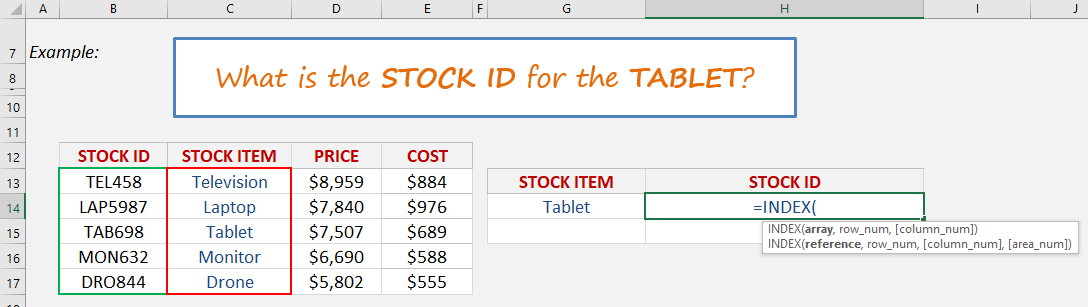STEP 2: The INDEX arguments:

## Array

Where is the list that contains the stock ids?

## =INDEX(B13:B17,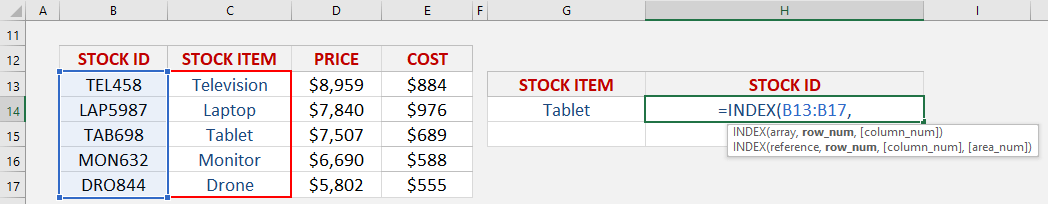## Row_num

What row number contains the data?

Let us use the Match function to get the row number.

## =INDEX(B13:B17, MATCH(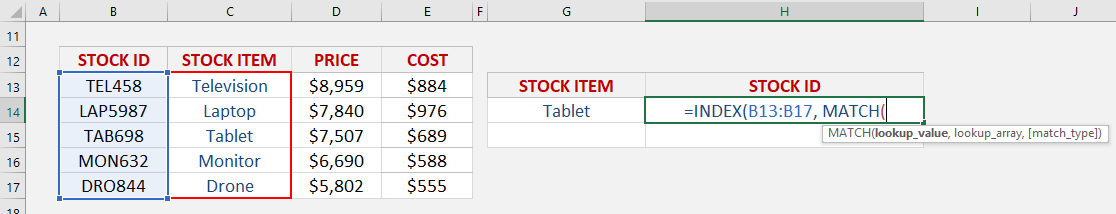STEP 3: The MATCH arguments:

## Lookup_value

What is the value that we want to match?

We want to match the Tablet.

## =INDEX(B13:B17, MATCH(G14,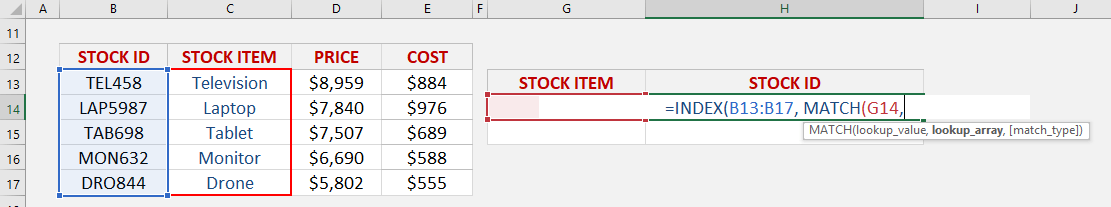## Lookup_array

Where is the list that contains the stock items?

## =INDEX(B13:B17, MATCH(G14, C13:C17,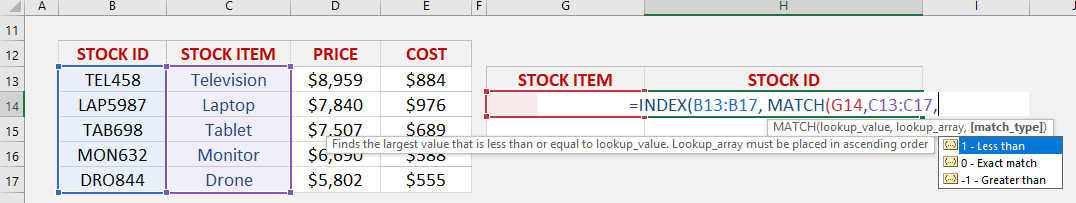## Match_type

What kind of matching do you want?

Let’s put in 0 to get the exact match

## =INDEX(B13:B17, MATCH(G14, C13:C17, 0))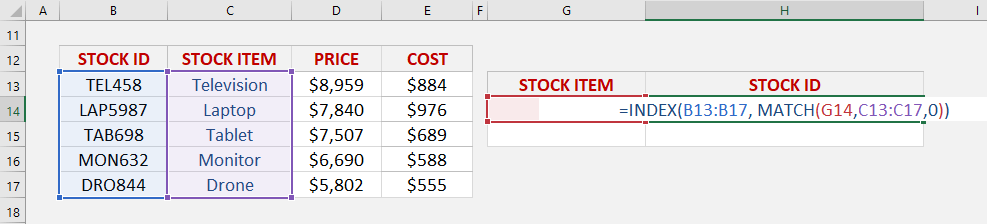With this, the MATCH function will get the row number containing the Tablet, which is row #3. Then with Row #3, we will get the stock id in that same row using the INDEX function.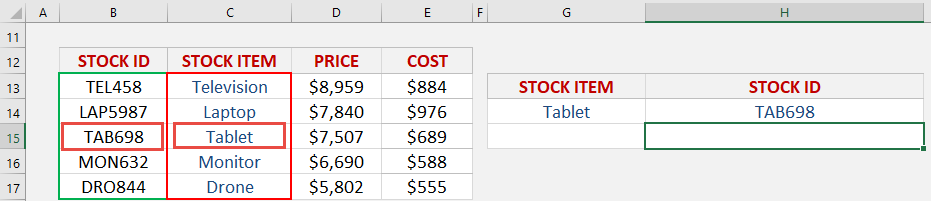How to Use the Index-Match Formula in Excel